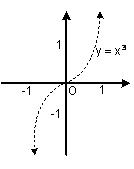×#### Thank you for registering.

One of our academic counsellors will contact you within 1 working day.

Click to Chat

1800-1023-196

+91-120-4616500

CART 0

• 0

MY CART (5)

Use Coupon: CART20 and get 20% off on all online Study Material

ITEM
DETAILS
MRP
DISCOUNT
FINAL PRICE
Total Price: Rs.

There are no items in this cart.
Continue Shopping```Definite Integration

Geometrical Interpretation of Definite IntegralIf f(x) > 0 for all x ∈ [a, b]; then ∫ba f(x) is numerically equal to the area bounded by the curve y = f(x), then x-axis and the straight lines x = a and x = b i.e.  ∫ba f(x)In general ∫ba f(x) dx represents to algebraic sum of the figures bounded by the curve

y = f(x), the x-axis and the straight line x = a and x = b. The areas above x-axis are taken place plus sign and the areas below x-axis are taken with minus sign i.e,

i.e. ∫ba f(x) dx area OLA – area AQM – area MRB + area BSCD

Note: ∫ba f(x) dx, represents algebraic sum of areas means, that if area of function y = f(x)

is asked between a to b.

=> Area bounded = ∫ba |f(x)|dx and not been represented by ∫ba f(x) dxe.g., If some one asks the area of y = x3 between -1 to 1.

Then y = x3 could be plotted as;

∴  Area = ∫0–1 –x3 dx + ∫10 x3 dx = 1/2

or, using above definition Area = ∫1–1 |x3| dx = 2 ∫10 x3 dx

= 2 [x4 / 4]10 = 1/2

But if, we integrate x3 between -1 to 1.

=>  ∫10 x3 dx = 0   which does not represent area.

Thus, students are adviced to make difference between area and definite Integral.

To read more, Buy study materials of Definite integral comprising study notes, revision notes, video lectures, previous year solved questions etc. Also browse for more study materials on Mathematics here.
```### Course Features

• 731 Video Lectures
• Revision Notes
• Previous Year Papers
• Mind Map
• Study Planner
• NCERT Solutions
• Discussion Forum
• Test paper with Video Solution